[BACK]Computer Modeling in Engineering & SciencesDOI: 10.32604/cmes.2022.018596

ARTICLE

Isogeometric Analysis with Local Adaptivity for Vibration of Kirchhoff Plate

College of Civil Engineering and Architecture, Key Laboratory of Disaster Prevention and Structural Safety of Ministry of Education, Guangxi Key Laboratory of Disaster Prevention and Structural Safety, Guangxi University, Nanning, 530000, China
*Corresponding Author: Sheng He. Email: hesheng@gxu.edu.cn
Received: 10 August 2021; Accepted: 30 August 2021

Abstract: Based on our proposed adaptivity strategy for the vibration of Reissner–Mindlin plate, we develop it to apply for the vibration of Kirchhoff plate. The adaptive algorithm is based on the Geometry-Independent Field approximaTion (GIFT), generalized from Iso-Geometric Analysis (IGA), and it can characterize the geometry of the structure with NURBS (Non-Uniform Rational B-Splines), and independently apply PHT-splines (Polynomial splines over Hierarchical T-meshes) to achieve local refinement in the solution field. The MAC (Modal Assurance Criterion) is improved to locate unique, as well as multiple, modal correspondence between different meshes, in order to deal with error estimation. Local adaptivity is carried out by sweeping modes from low to high frequency. Numerical examples show that a proper choice of the spline space in solution field (with GIFT) can deliver better accuracy than using NURBS solution field. In addition, for vibration of heterogeneous Kirchhoff plates, our proposed method indicates that the adaptive local h-refinement achieves a better solution accuracy than the uniform h-refinement.

Keywords: Isogeometric analysis; local refinement; adaptivity; vibration; kirchhoff plate

1  Introduction

Isogeometric analysis (IGA) was proposed in  to assemble analysis in Computer Aided Engineering (CAE) and Computer Aided Design (CAD). Due to high continuity of NURBS basic functions [1,2], NURBS-based IGA is widely used in the field of engineering structure, such as shape optimization of structure , vibration of plates, including Kirchoff plate  and Reissner–Mindlin plate . These studies have shown that IGA results are often better than traditional finite element method (FEM) based approaches. Since for d ≥ 2, NURBS are defined with tensor product form, the refinement is constrained by the global structured grid (see Fig. 1a). Unfortunately, this leads to extra computational costs as the mesh is refined for the solution field. Moreover, the tensor product based refinement does not facilitate local refinement to capture local phenomenon, e.g., sharp gradients or boundary layer.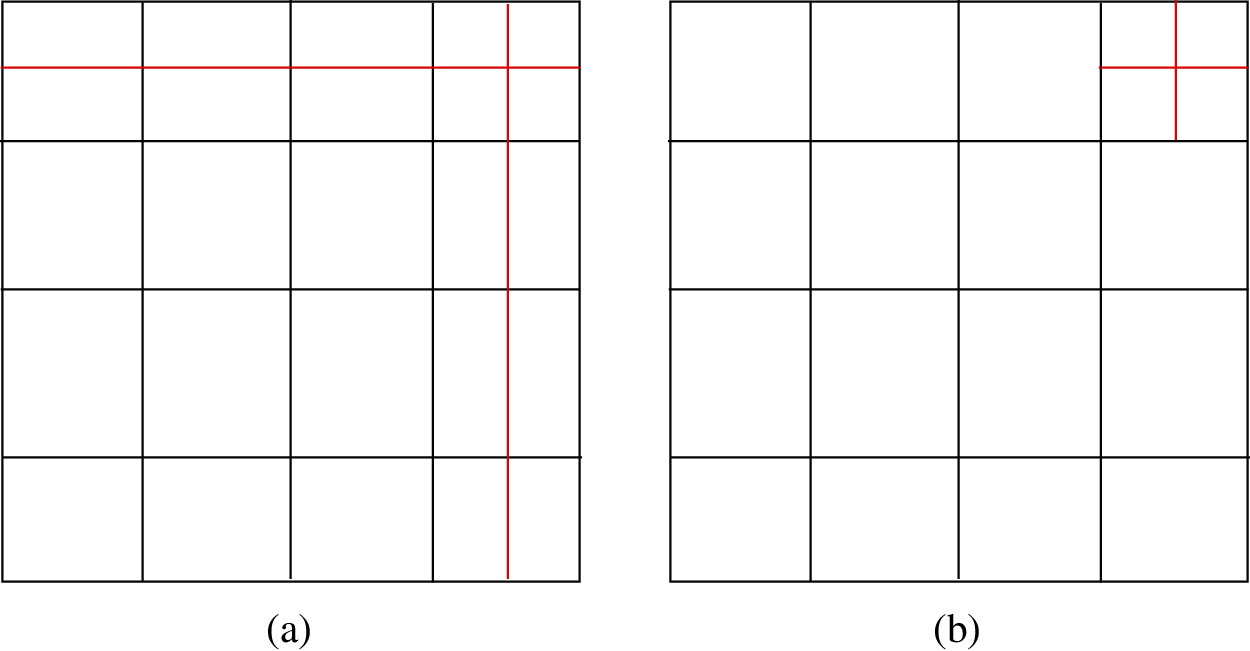Figure 1: (a) NURBS global refinement and (b) expected local refinement

The organization of this paper is as follows. In Section 2, the variational form of linear elasto-dynamics, based on Kirchhoff plate theory, is set up. The weak form, based on IGA and GIFT framework, is introduced in Section 3. In Section 4, a-posteriori error estimation and the hierarchical local refinement process is developed. In Section 5, the a-posteriori error estimates and hierarchical local refinement of proposed in Section 4 is combined with MAC for modal analysis, and the resulting error-driven local adaptivity for vibration is presented. In Section 6, several numerical examples are presented. These results using GIFT approach show two major achievements: (1) Despite a poor geometric parameterization, an accurate numerical approximation can be obtained by adopting an appropriate parameterization in solution field. (2) When structural vibration is localized, the proposed adaptive refinement delivers a better convergence rate than the uniform refinement.

2  Problem Statement

Let ΩRd represent the spatial domain of an elastic plate, and let (x, y, z) denote the Cartesian coordinate system. Moreover, let (u, v, w) denote the deflections of the plate in the (x, y, z) directions, respectively, and h denote the thickness of the plate in the z direction. Based on the Kirchhoff plate theory, see e.g., , the displacement components u and v, at a distance z from the neutral surface, can be expressed as

u=zwx,v=zwy,

where w is the deflection of the neutral plane of the plate in the z-direction. Thereby, w is the only independent variable, and we obtain a simple relationship

u:={u,v,w}T={zx,zy,1}Tw.(1)

Moreover, the relationship between the three components of strain and the deflection is given by

ε:={εxx,εyy,γxy}T=z{2x2,2y2,22xy}Tw.(2)

By introducing the differential matrices

H:={zx,zy,1}T,E:={2x2,2y2,22xy}T,(3)

the relations (1) and (2) can be written as

u=Hw,ε=zEw.(4)

Let C be the matrix of material stiffness constants. The in-plane (normal and shear) stresses σxx, σyy, and σxy can then be obtained, by substituting the value of ε into the constitutive relation σ=Cε, as follows:

σ=zCEw.(5)

To evaluate the strain at the neutral plane of the plate, hence independent of the coordinate z, we introduce the pseudostrain εp, which is defined as

εp=Ew.(6)

Let the parameter D = Eh312(1 − ν2) denote the bending stiffness of the plate, where E is the Young's modulus, and ν is the Poisson's ratio. Furthermore, let Mxx, Myy, and Mxy denote the bending moments, and twisting moments, respectively, which are defined as

Mxx=D(2wx2+ν2wy2),Myy=D(ν2wx2+2wy2),Mxy=D(1ν)2wxy.(7)

These three components of the moments then define the pseudostress as

σp={Mxx,Myy,Mxy}T.(8)

Let D denote the constant matrix of the material property and the plate thickness, which is defined as

D=D[1ν0ν10001ν2].(9)

For a thin plate, the generalized Hooke's law then gives the relation of pseudostress and pseudostrain as

σp=Dεp.(10)

Now let Vxz and Vyz denote the shear forces. Considering the moment equilibrium of the plate cell with respect to the x- (and y-) axis, and neglecting the second order small terms, leads to a relation in terms of moments

Vxz=Mxxx+Mxyy,Vyz=Mxyx+Myyy.(11)

Let ρ denote the mass density of the plate material. The plate cell is then subjected to the inertial force ρhw¨. In deriving the system equilibrium equations, now consider the equilibrium of the small plate cell in the z direction, which can be written as

dVxzdy+dVyzdx+(bzρhw¨)dxdy=0,

where bz is the external force. Using d Vxz = ∂ Vxz∂ x dx, and d Vyz = ∂ Vyz∂ y dy, we get

Vxzx+Vyzy+bz=ρhw¨.

Substituting the relations of shear forces from (11), and the moments from (7), we get the following equation for homogeneous and isotropic plates

D(4wx4+24wx2y2+4wy4)+ρhw¨=bz.(12)

D(4wx4+24wx2y2+4wy4)+ρhw¨=0.(13)

We consider the clamped boundary conditions on all side, i.e.,

w=wn=0,on Ω.(14)

We now introduce the function space V0 = {vH2(ω) : v = ∂ v∂ n = 0 on γ }. Then the elasto-dynamic vibration problem in variational form reads [7,28]

Ωρu~Tu¨dΩ+Ωε~pTσdΩ=0,(15)

where u is the displacement field, u~ is the virtual displacement, and ε~ is the virtual strain. Then, using the relation of pseudostress and pseudostrain (10), the weak form (15) can be rewritten as

Ωρ(Hw~)T(Hw¨)dΩ+Ω(Ew~)TD(Ew)dΩ=0,w~V0.(16)

3  The Discrete Form Using GIFT

Let P be the parametric domain. The physical domain ω is parametrized on P by a geometrical mapping F

F:PΩ,x=F(ξ),xΩ,ξP.(17)

We assume that the domain ω may consist sub-domains such that Ω=i=1NΩi. Typically, the geometrical map F is given by a set of basis functions Ni1, i2, …, id(ɛ) and a set of control points P i1, i2, …, id as

F(ξ)=i1=1n1i2=1n2id=1ndPi1,i2,,idNi1,i2,,id(ξ),(18)

where Ni1, i2, …, id(ɛ) can be a d-dimensional tensor product of NURBS, B-splines, T-splines, PHT-splines, etc. For brevity reasons, we introduce two sets of multi-indices (i1, i2, …, id) of NURBS basis functions by

I={(i1,i2,,id):i1{1,,n1},,id{1,,nd}}(19a)

J={(i1,i2,,id):i1{1,,m1},,id{1,,md}}.(19b)

Moreover, wherever suitable, for multi-index (i1, i2, …, id) we will interchangeably use the collapsed notation k Thence, Eqs. (17) and (18) are written as

x(ξ)=kIPkNk(ξ).(20)

In what follows, we will refer to the set {Nk()}kI as the geometry basis. For change of variables, we will also need the Jacobian matrix J(ɛ) of the mapping F, which is given by

Jij(ξ)=xiξj(ξ)=kIPkiNk(ξ)ξj.(21)

The GIFT method is presented in detail by Atroshchenko et al. . In IGA, the solution field uI is represented through the same spline functions which are used for the geometry

uI(ξ)=kINk(ξ)UkI,(22)

where UkI are unknown control variables. In GIFT, we depart from classical IGA by choosing a solution basis {Mk()}kJ, which is possibly different from the geometry basis. As with {Nk()}kJ, the basis {Mk()}kJ, can also be a tensor product of NURBS, B-splines, T-splines, PHT-splines, etc. Thereby, we look for the solution u G, possibly independent of geometry, as follows:

uG(ξ)=kJMk(ξ)UkG,(23)

where UkG are unknown control variables. Note that, if basis functions are chosen as {Ml(ϵ)}kJ={Nk(ϵ)}kI, then the framework is based on IGA. To approximate the unknown variable in the physical space, we introduce the spline space VG as follows

VG={uh:uhspan{Mk(ξ)F1}}.(24)

Now, using the basis functions M k( ), we approximate the deflection w in Eq. (16) as

w=kJMk(ξ)wk(25)

where M k(ɛ) are basis functions, and w kare unknown control variables. Substituting (25) in (16), we obtain the discrete form of the dynamical equation as follows:

w~T(Kw+Mw¨)=0,(26)

where w denotes the vector of deflections at the control points, and K and M respectively denote the stiffness and mass matrices, which are defined as follows

K=Ω(EMx)TD(EMx)dΩ,(27a)

M=Ωρ(HMx)Tm(HMx)dΩ,(27b)

where M xdenotes the basis functions from the space VG, and the matrix m the mass matrix

m=[h312000h312000h].(28)

The general solution of the free vibration Eq. (26) can be expressed as

w(t)=ϕexp(iλt),(29)

where i is the imaginary unit, is the eigenvector, and λ is the natural frequency. Substituting (29) into (26), and ignoring the virtual quantity, the natural frequency λ of thin plates can be calculated by solving the following generalized eigenproblem:

(Kλ2M)ϕ=0.(30)

3.1 Boundary Conditions

As eigenproblem (30) does not include the essential boundary conditions, it is necessary to find a proper way to impose essential boundary conditions. Some of the methods proposed in the literature to impose essential boundary conditions, e.g., [29,30] can be extended to the isogeometric framework. However, for efficiency reasons, we prefer another approach .

In this approach, simply supported boundary condition can be imposed through fixing the z-component of the first row of control variables for the respective boundary. Clamped boundary conditions can be imposed by fixing the z-component of the first two rows of control variables for the respective boundary.

In the next section, we introduce the error in the energy norm, and then define the hierarchical local refinement process. Unless otherwise stated, in what follows, we only compute the energy norm of the variables.

4  Hierarchical Local Refinement

For the complex cases, it is difficult to obtain the analytical solution for generalized eigenproblem (30). Therefore, to compute the errors in our numerical solution, we compute the solution on a refined mesh. This mesh is called the refined mesh, and the solution on it is called the better solution. Let u¯ denote the better solution, and u h denote the numerical solution at a mesh with characteristic mesh size h. Then, the error in the numerical solution can be written as e=u¯uh. Note that the refined mesh elements are created by dividing each element of the current mesh into Ne = 2dLeelements, where d is the dimension of the problem, and Le is the level of refinement, which is set by the user. The element-wise error in the energy norm for the ith element Ωie is then defined as

||e||Ωie2=Ωie(ε¯εh)TD(ε¯εh)dΩie.(31)

Now Let N denote the total number of elements in the domain. Then, the energy norm of error in the whole domain is defined as

||e||2=i=1N||e||Ωie2.(32)

Let η denote the error tolerance, i.e., if the error in any element is above this threshold, then the element is marked for refinement. For element marking, we employ the mean-value strategy with some simple modification. To be specific, we first select the elements for which the error satisfies ||e||Ωie2>τ||e||2, where τ is certain percentage (chosen as 20% in this article). Let us assume that the number of such selected elements be ||e||Ωie2. Out of these elements, let the number of elements with largest error N¯ be NL, then remove these NL elements, and subsequently take mean value of error for the rest of the elements, and compute the new tolerance η as follows

η=1N¯NLi=1N¯NL||e||Ωie2.(33)

Thence, we mark the elements where ||e||Ωie2>η, and refine them into Ne elements. The process of adaptive local refinement has been summarized in Algorithm 1. For the 2D case, with Le = 1, the adaptive process of Algorithm 1 is presented in Fig. 2.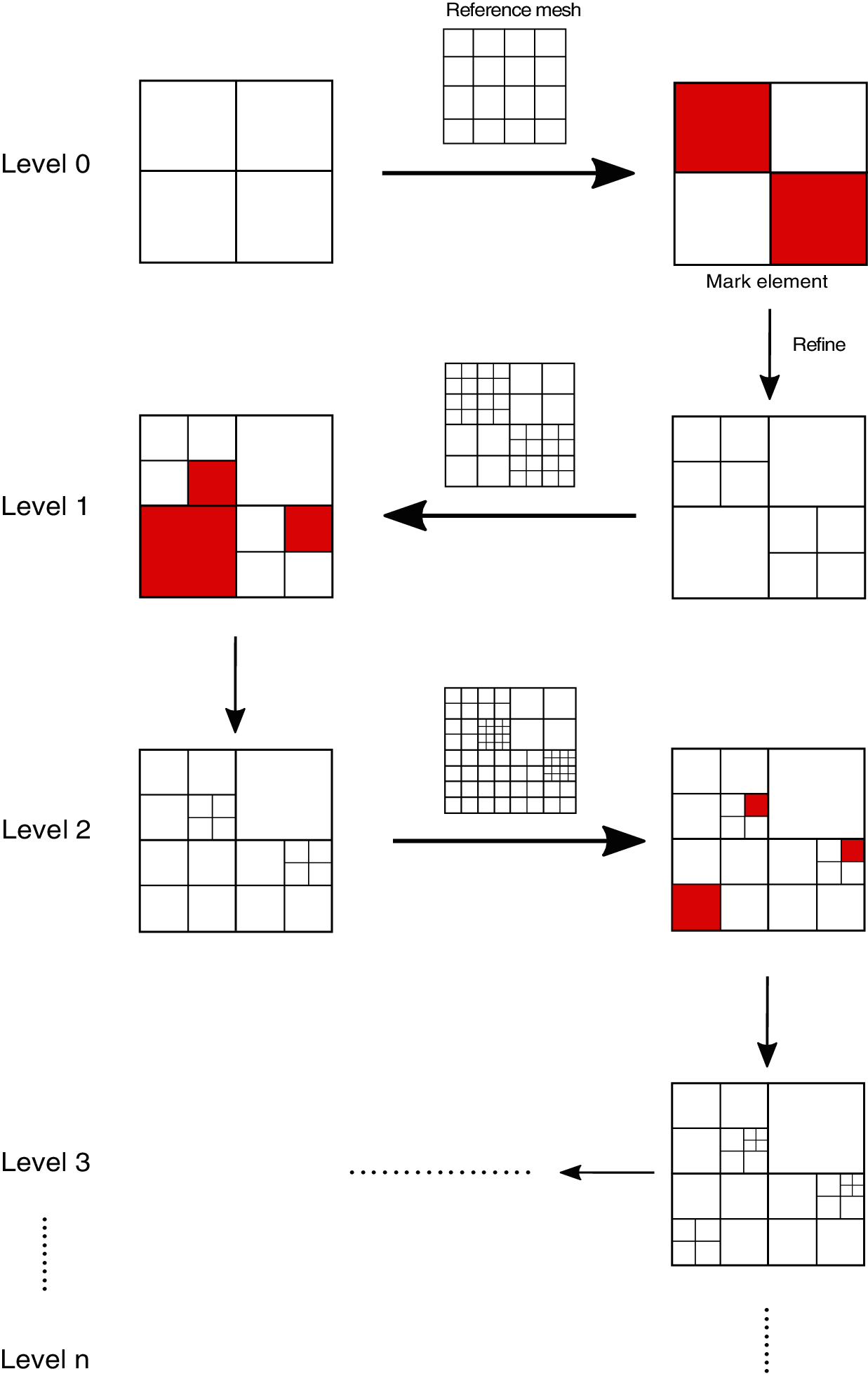Figure 2: Adaptive refinement process of Algorithm 1 in 2D

5  Error-Driven Local Adaptivity for Vibration

In this section, error-driven local adaptivity based on the Algorithm 1 combined with MAC for vibration is presented. The purpose of our adaptive local refinement method for vibration is to get more accurate solution by less computing resources. Supposed that eiλ and eiϕ is the error indicator of natural frequency and eigenvector, respectively, at ith mode defined by

eiλ=|λ~i~λihλ~i~|,eiϕ=ϕ~i~ϕihϕ~i~,(34)

wherein λ~i~ means natural frequency at i~th mode in refined mesh and λi is natural frequency at ith mode in the current mesh. An issue that has to be taken into consideration is how to construct the relationship between i and i~, that is to say, if λi in current mesh is expected to be optimized, how could we find the related λ~i~ in the refined mesh to compute eiλ. In order to resolve this problem, MAC is introduced in the following section.

5.1 Modal Assurance Criterion

Modal Assurance Criterion (MAC) is a statistical indicator originally proposed for orthogonality check  and has been developed as one of the most well-known method to compare modal vectors quantitatively . In this paper, it is utilized for assistance for error estimation between two different mesh systems. The value of MAC is computed as the scalar product of the two sets of normalized eigenvectors ϕih and ϕ~i~, where ϕi~h the is eigenvector at the i~th mode in the refined mesh, and ϕih is the eigenvector at the ith mode in current mesh. Note that, unless otherwise stated, the eigenvectors are normalized. The outcome will be assembled into MAC matrix M using the formula

Mi~,i(ϕ~i~,ϕih)=Ωϕ~i~TmϕihdΩϕi~m2ϕihm2,(35)

where ||⋅||m is the mass norm and defined by

m:=[Ω()Tm()dΩ]12,(36)

and m is the mass matrix defined in Eq. (28). The values of the MAC matrix are located in the interval [0, 1], where 0 means no consistent resemblance whereas 1 means a consistent correspondence. Generally, it is accepted that large values denote relatively consistent correlation whilst smaller value represents poor association of the two modal vectors. For instance, an example of MAC matrix M are illustrated in Table 1 with 3D view in Fig. 3a. For the first three modes, it is obvious that ϕ1h,ϕ2h,ϕ3h are correlated to ϕ~1,ϕ~2,ϕ~3 respectively but orthogonal to those in rest modes. However, when it comes to the 4th and 5th modes, we can see the resemblance are not unique any more. Instead, it is exhibited as a block made up with 4 bars marked with red circle in Fig. 3a. That is because 4th and 5th modes in both current and refined domains are multiple modes that ϕ4h,ϕ5h and ϕ~4,ϕ~5 separately represent one pair of basis for eigenvector space for current and refined mesh. The method to tackle with the multiple modes is introduced in our work , and after the measurement, it can be seen that the block of MAC matrix shown in red circle in Fig. 3a are merged into a bar in red circle in Fig. 3b, namely, [M4,4,M4,5M5,4,M5,5]M45,45. Accordingly, the conversion is also made in Table 2. MAC helps to construct correlation of modal vectors between current and refined domain. Combined with scheme of error estimation and hierarchical refinement proposed in Algorithm 1, strategy of adaptive local refinement for vibration would be developed in the following section.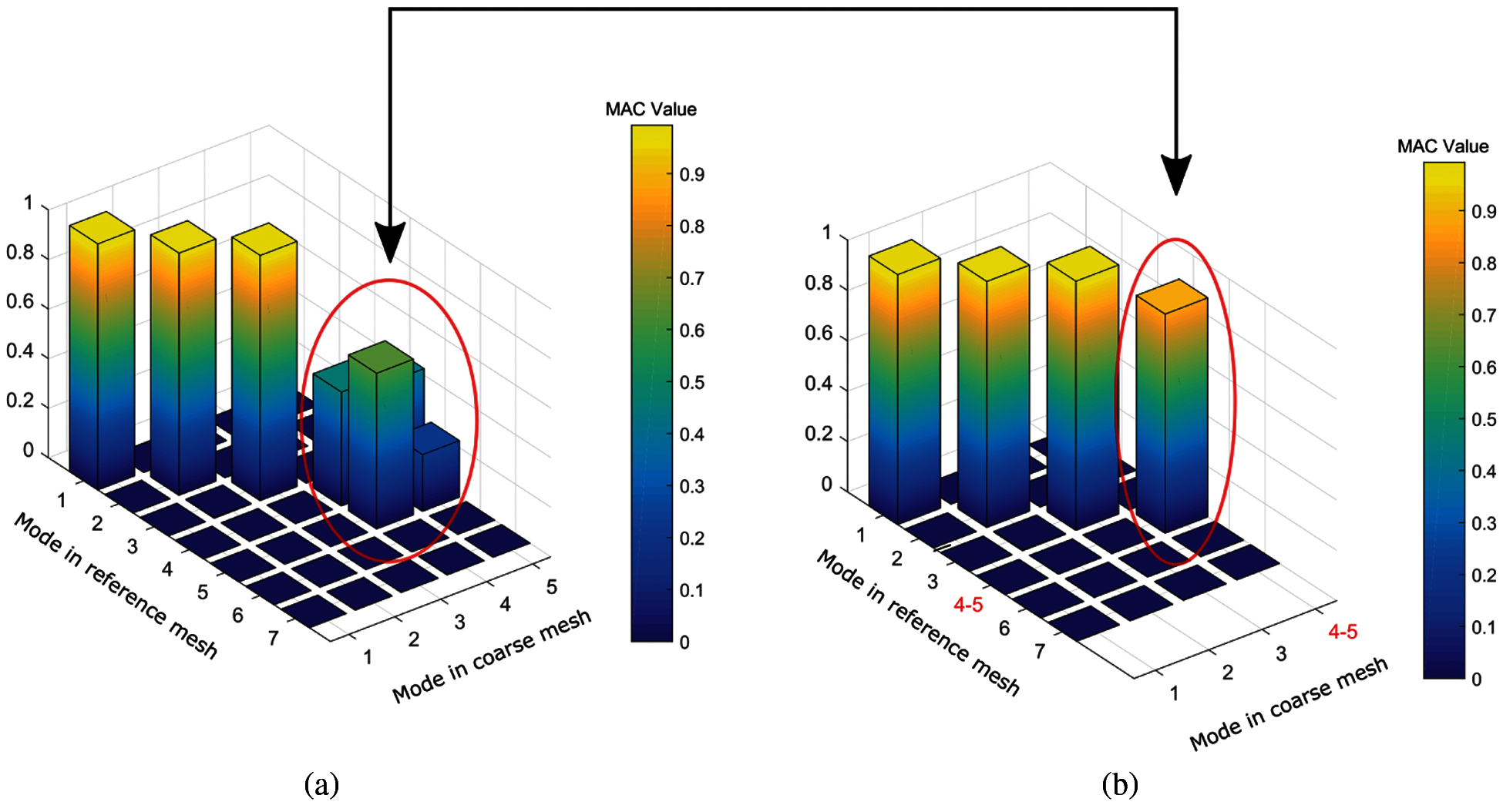Figure 3: An example of 3D view for MAC values. (a) MAC matrix with multiple eigenvectors (in red circle) before projection; (b) MAC matrix after projection of multiple eigenvectors (in red circle)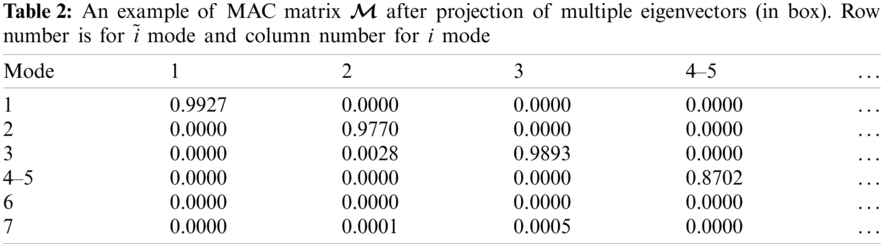5.2 Adaptive Local Refinement for Vibration

As we know, mode shapes of structural vibration are often different from modes to modes. One may pay attention to the mode shapes around specific range of frequency according to engineering problems. For general applications, in this paper, the adaptive refinement is carried out via sweeping modes from low to high frequency. The procedure is summarized in Algorithm 2.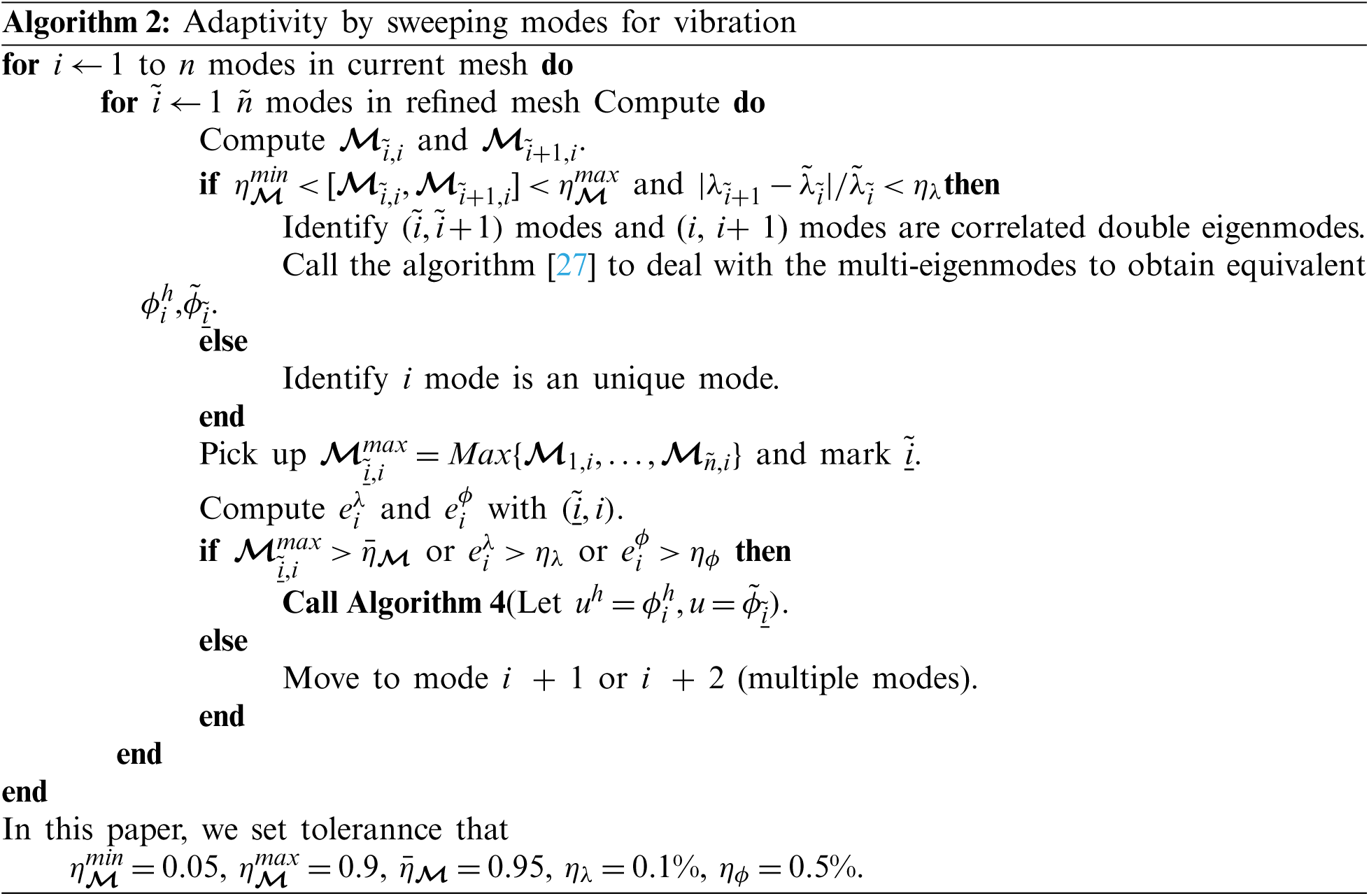6  Numerical Tests

Four numerical examples are carried out for the following purposes. Example in Section 6.1 is the vibration investigation of circular plate to show that GIFT method has the merit of flexibility to choose spline space in solution field independently from that of geometry, which would yield better solution compared to IGA on condition that control points describing geometry are distributed unreasonably. Examples in Sections 6.26.4 employ GIFT, with NURBS representing geometry and PHT describing solution field, to study vibration problem for heterogeneous plate with different shapes, especially, analysis of multiple modes is presented in example in Section 6.2. These heterogeneous plate perform obvious concentrated local response where PHT is able to show the advantage of local refinement. Noted that in instances of Sections 6.26.4, due to lack of theoretical solution for the problems, we adopt the computational result from the very fine uniform mesh as the approximation of analytical solution.

6.1 Circular Plate

The geometric and material parameters for circular plate are listed in Table 3, with radius R, thickness h, Young's modulus E, and density ρ. On assumption that the geometry of circular plate is established by cubic NURBS basis functions with irregularly distributed control points 24 × 24, shown in Fig. 4a. In IGA scheme, the solution space in Fig. 4b is imposed to be the same as geometric space. However, GIFT is free to pick up the solution space so that the uniform one in Fig. 4c is preferred here. For better comparing with theoretical results , the normalized natural frequency related parameter λ¯N is defined

λ¯N=(λR2Dρh)12,(37)

where λ is natural frequency and D is flexural stiffness. Consequently, supposed that λ¯Nh is computational natural frequency related parameter, the relative error eN can be written as

eN=|λ¯Nλ¯Nh|λ¯N.(38)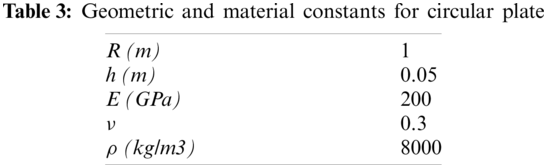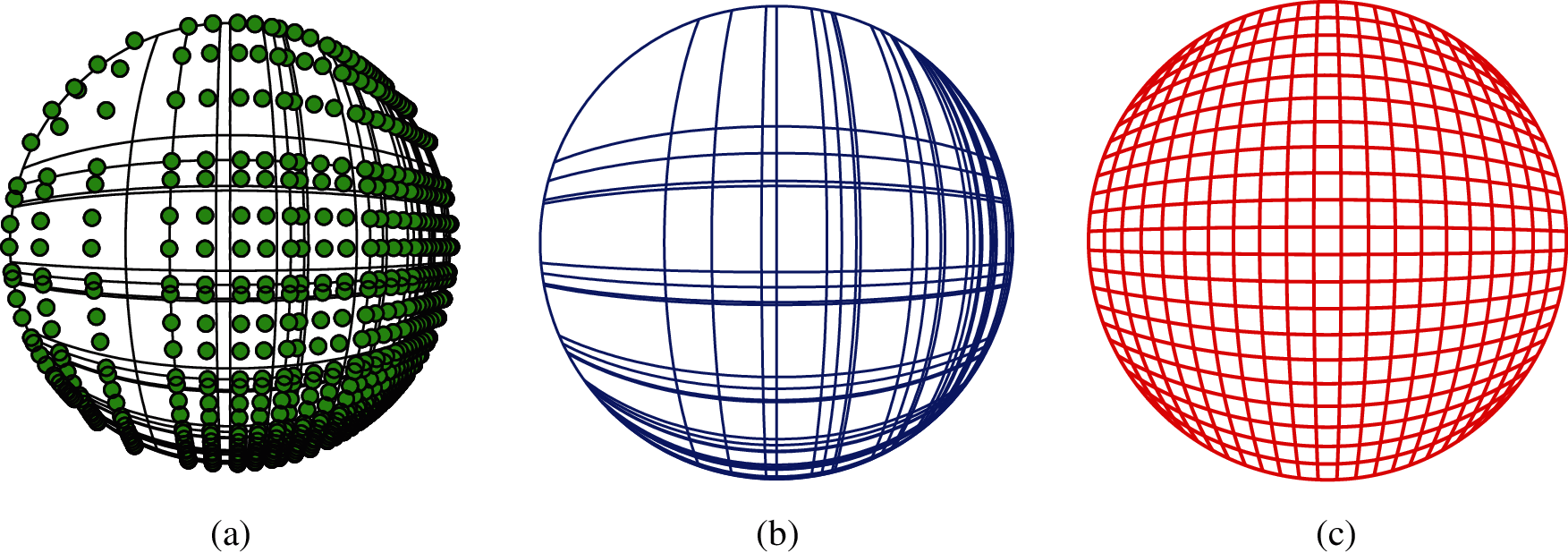Figure 4: The same geometry parameterization and different spline spaces in solution field of IGA and GIFT for circular plate. Cubic NURBS for both IGA and GIFT. (a) Geometry with 24 × 24 control points; (b) Geometry with 24 × 24 control points; (c) Geometry with 24 × 24 control points

In this example, IGA and GIFT method is compared with exact solution in simply supported boundary condition and clamped boundary condition separately, where the difference of these two boundary conditions has been clarified in Section 3.1. The results are illustrated in Tables 4, 5 and Fig. 5. It is observed that GIFT shows a better accuracy than IGA with increasing of modes. This example inspires us that when the geometric space of structure is not good enough, we can count on GIFT to re-construct the solution space to achieve a better precision.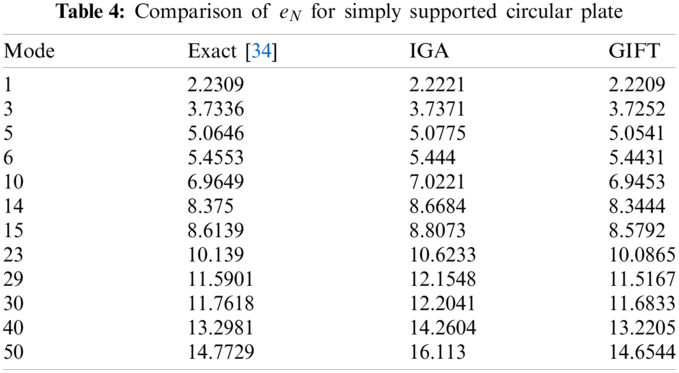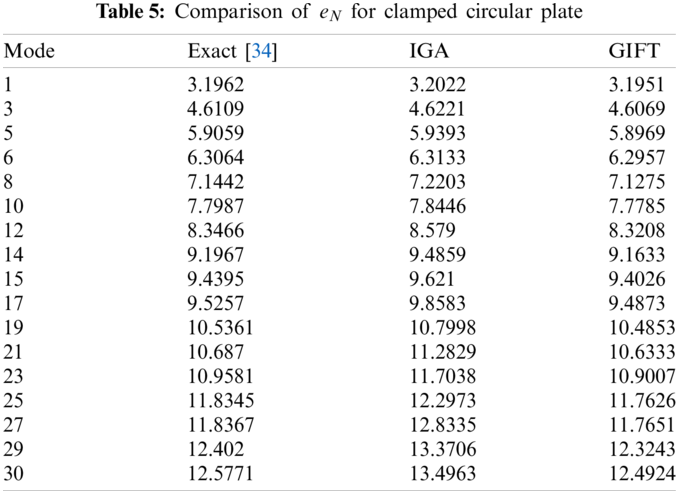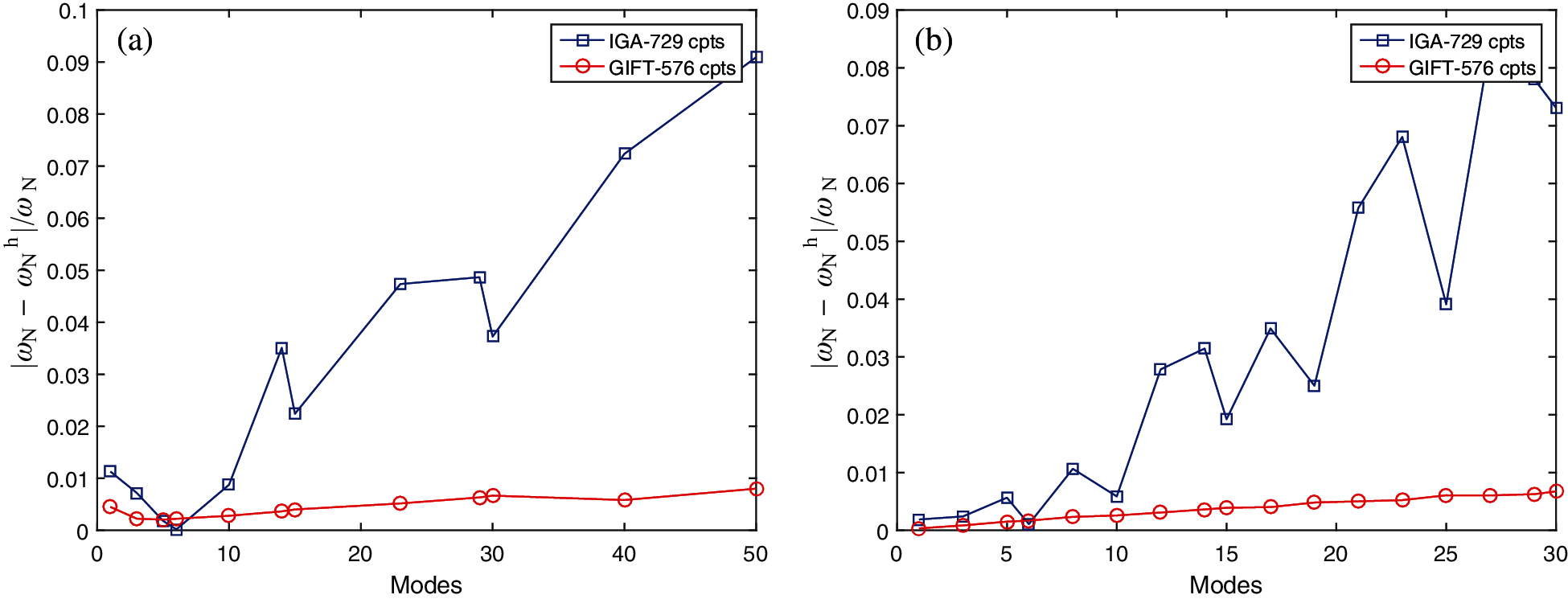Figure 5: Comparison of eN between IGA (729 control points) and GIFT (576 control points) in solution field (Cubic NURBS, cpts control points). (a) Simply support; (b) clamped

6.2 Heterogeneous L-Shape Plate

In this test, a heterogeneous L-shape structure is built with 3 Patches where relevant geometric parameters and material constants are displayed in Fig. 6a. The whole domain boundary is simply supported. Density ρ is 2700, and thickness h is 0.01 and Poisson's rate ν is 0.3. The Young's modulus of 3 Patches is E2 = E = 107, E1 = E3= 1000E2 respectively so that it is evident the vibration would be concentrated in the Patch 2 as the material is softest in this area. As explicitly discussed on the principle in Section 5, the adaptivity starts with an initial mesh in Fig. 7a from the 1th mode and afterwards sweeps from low to high frequency. Here we would like to emphasize that firstly, the error indicators of both natural frequency e1λ and eigenvector e1ϕ at 1th are compared in the situation that the refined mesh is generated with refinement level Le = 1, 2, 3, respectively. Seen from Figs. 9a and 10a, the three plots perform the almost same convergence rate, which symbolizes the refinement level Le = 1 we select for error estimation is reasonable. In addition, the adaptive performance in different modes is able to be fairly understood from Figs. 7, 9b and 10b. Particularly, from Fig. 9b, we can see the local refinement is conducted at 1th, 2–3th, 5–6th, 9–10th modes. While there is no refinement for 4th, 7–8th, 11th, 12–13th, 14–15th since the solutions by mesh state which they inherit from the previous modes are underneath the error indicator, namely, it does not meet the condition at Step (h) in Algorithm 5.2 and then move to Step (i). Similarly, this state can be also observed in Fig. 7 that 2–3th mode share the mesh with 4th mode, and 5–6th mode share the mesh with 7–8th mode, etc. Furthermore, it is noted that in some situation that eiλελ in Fig. 9b, though, it still has to be refined. That is because condition eiϕεϕ is unsatisfied. Obviously, from Figs. 9b and 10b, compared with eiλ, the decrease of eiϕ is more tough to achieve. Besides, the contrast of relative error in energy norm is made between adaptive refinement eadp and uniform refinement euni, where the errors are calculated by comparing to the approximation of exact solution u^ thatFigure 6: A simply supported heterogeneous L-shape plate: (a) geometric parameters and (b) discretization of patches, E2=E,E1=E3=1000E2Figure 7: Adaptive refinement process of 1–15th mode for vibration of L-shape plate. Especially, 2–3th, 5–6th, 7–8th, 9–10th, 12–13th, 14–15th modes are multiple modes. Geometry-NURBS with order p1 = 1, p2 = 1; Solution Field-PHT with order q1 = 3, q2 = 3. (a) Initial mesh; (b) Adaptive mesh for 1th mode; (c) Adaptive mesh for 2–3, 4th mode; (d) Adaptive mesh for 5–6, 7–8th mode; (e) Adaptive mesh for 9–10, 11, 12–13, 14–15th mode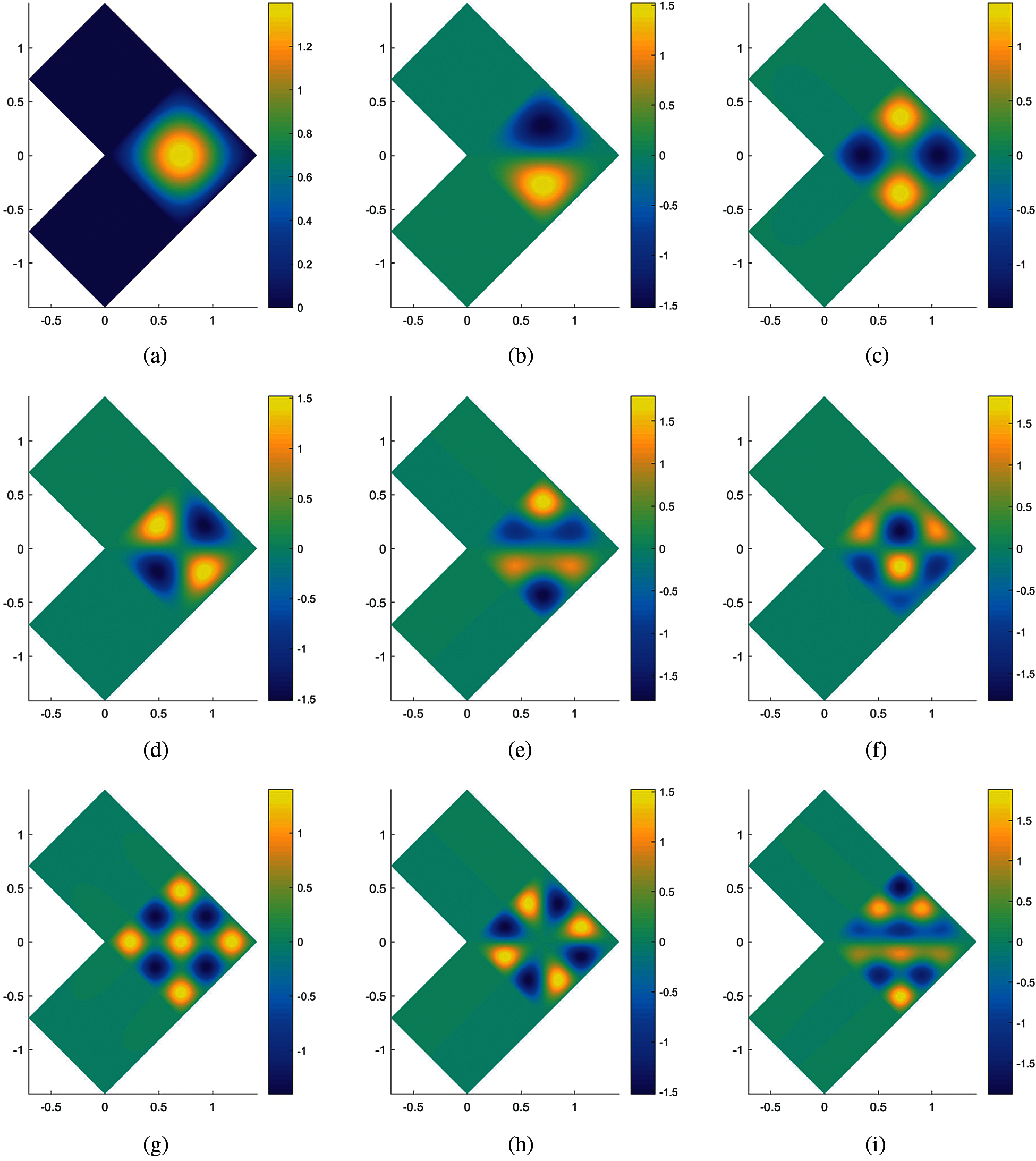Figure 8: Mode shapes 1–15th of L-shape plate, where λNi is normalized natural frequency at mode i, calculated by λNi=λi/λ1,i=1,2,3n. (a) 1th mode,λN1=1; (b) 23th mode,λN23=2.50; (c) 4th mode,λN4=4.00; (d) 56th mode,λN56=4.99; (e) 78th mode,λN78=6.50; (f) 910th mode,λN910=8.45; (g) 11th mode,λN11=9.00; (h) 1213th mode,λN1213=10.00; (i) 1415th mode,λN1415=12.50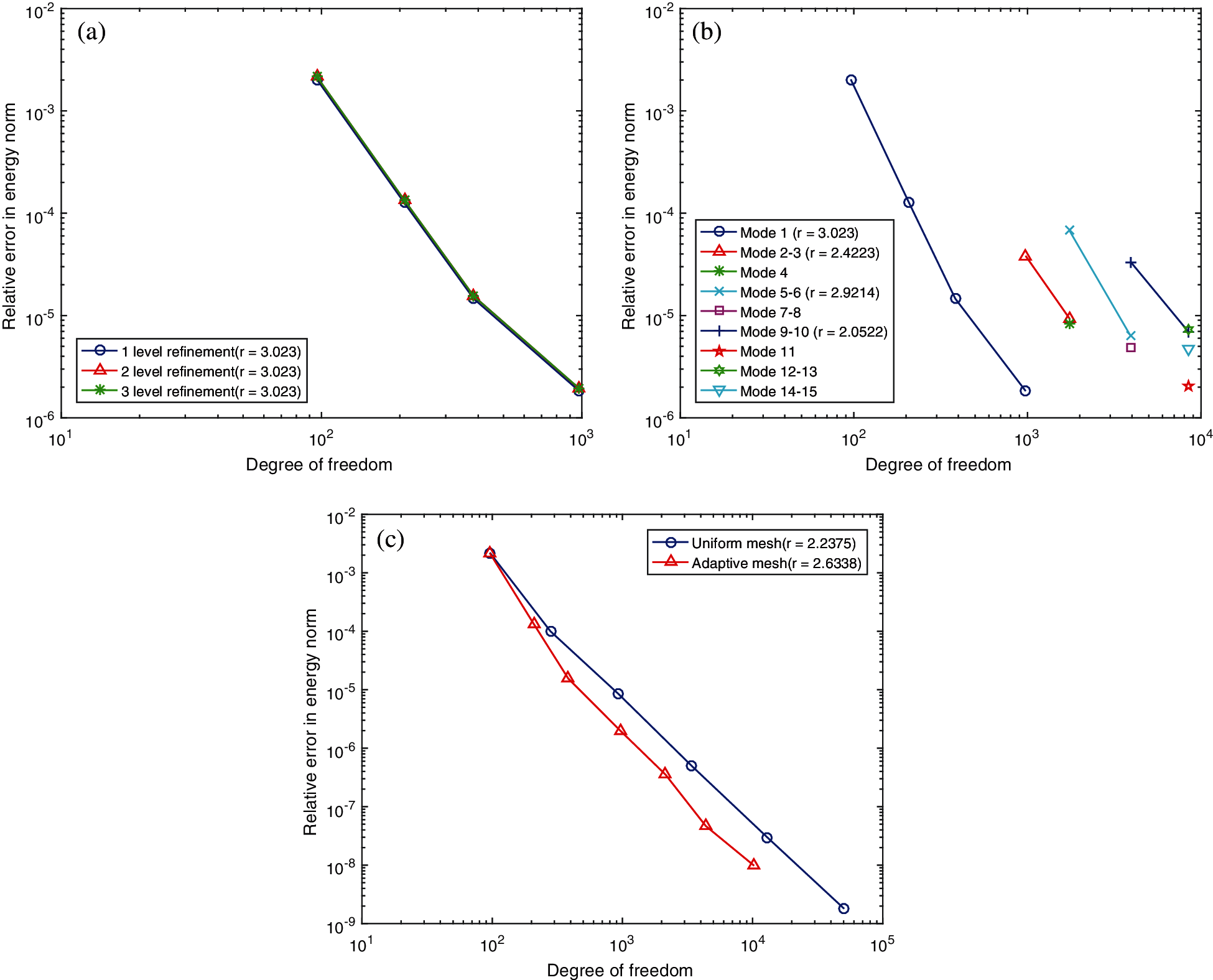Figure 9: (a) Comparisons of error indicator of natural frequency at 1th mode e1λ among refinement level of Le= 1, Le = 2, Le = 3; (b) error indicator of natural frequency of 1–15th mode during adaptive refinement process by sweeping modes; (c) contrast of relative error of natural frequency in energy norm at 1th mode between adaptive refinement and uniform refinementFigure 10: (a) Comparisons of error indicator of eigenvector at 1th mode e1ϕ among refinement level of Le = 1, Le = 2, Le = 3; (b) error indicator of eigenvector of 1–15th mode during adaptive refinement process by sweeping modes; (c) contrast of relative error of eigenvector in energy norm between adaptive refinement and uniform refinement

6.3 Heterogeneous Plate with a Hole

In this section, a plate with a hole where the boundary simply supported is shown in Fig. 11, consisting of two material Patches with E1 = E = 109, E2 = 100E1. Density ρ is 2700, and thickness h is 0.01 and Poisson’s rate ν is 0.3. Consequently, the vibration response and local adaptive refinement is undoubtedly centralized in the Patch 2 area, seen in Figs. 12 and 13. Different from L-shape example in Section 6.2, the geometry of the hole is non-linear so that cross-derivative of the parametrization in Eq. (16) are non-zero, which is a good opportunity to validate our algorithm is available in terms of non-linear mapping as well. The convergence rate plot of error indicators in both of Figs. 14a and 15a support the reasonability of adopting refinement level Le = 1 for reference parametrization in solution field. Without any surprise, adaptive refinement performs a higher convergence rate than uniform refinement, based on an approximation of solution by a very fine mesh with 132,870 dof.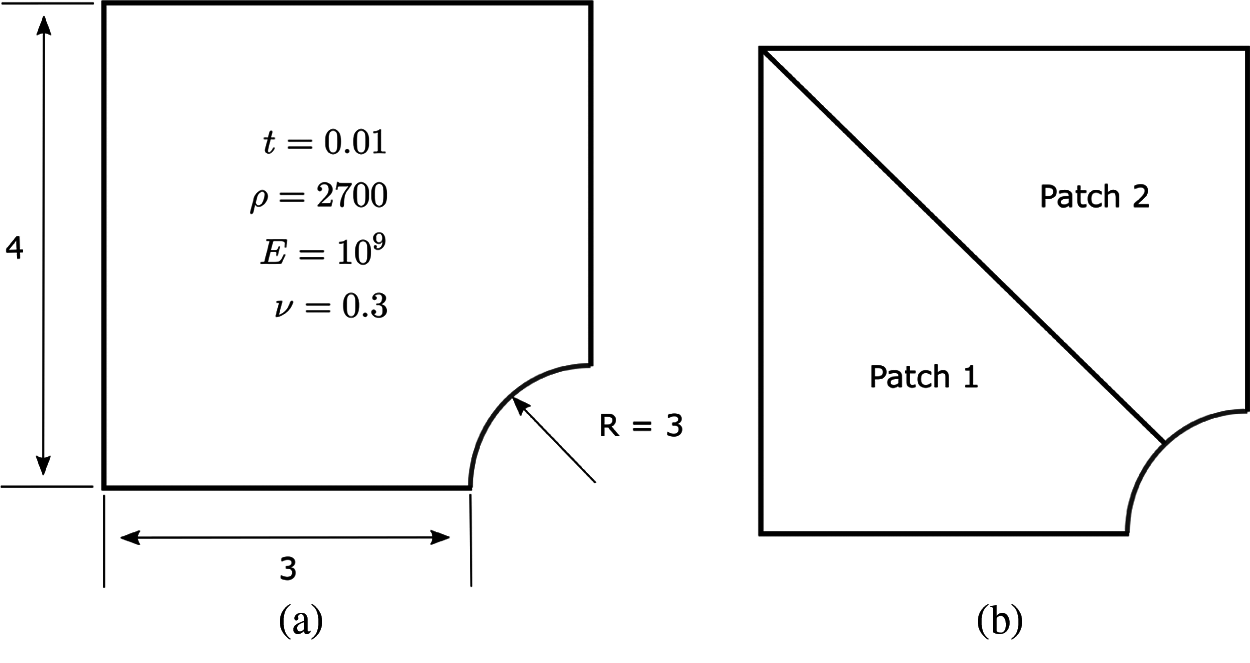Figure 11: A simply supported heterogeneous platehole: (a) geometric parameters and (b) discretization of patches, E1 = E, E2 = 100E1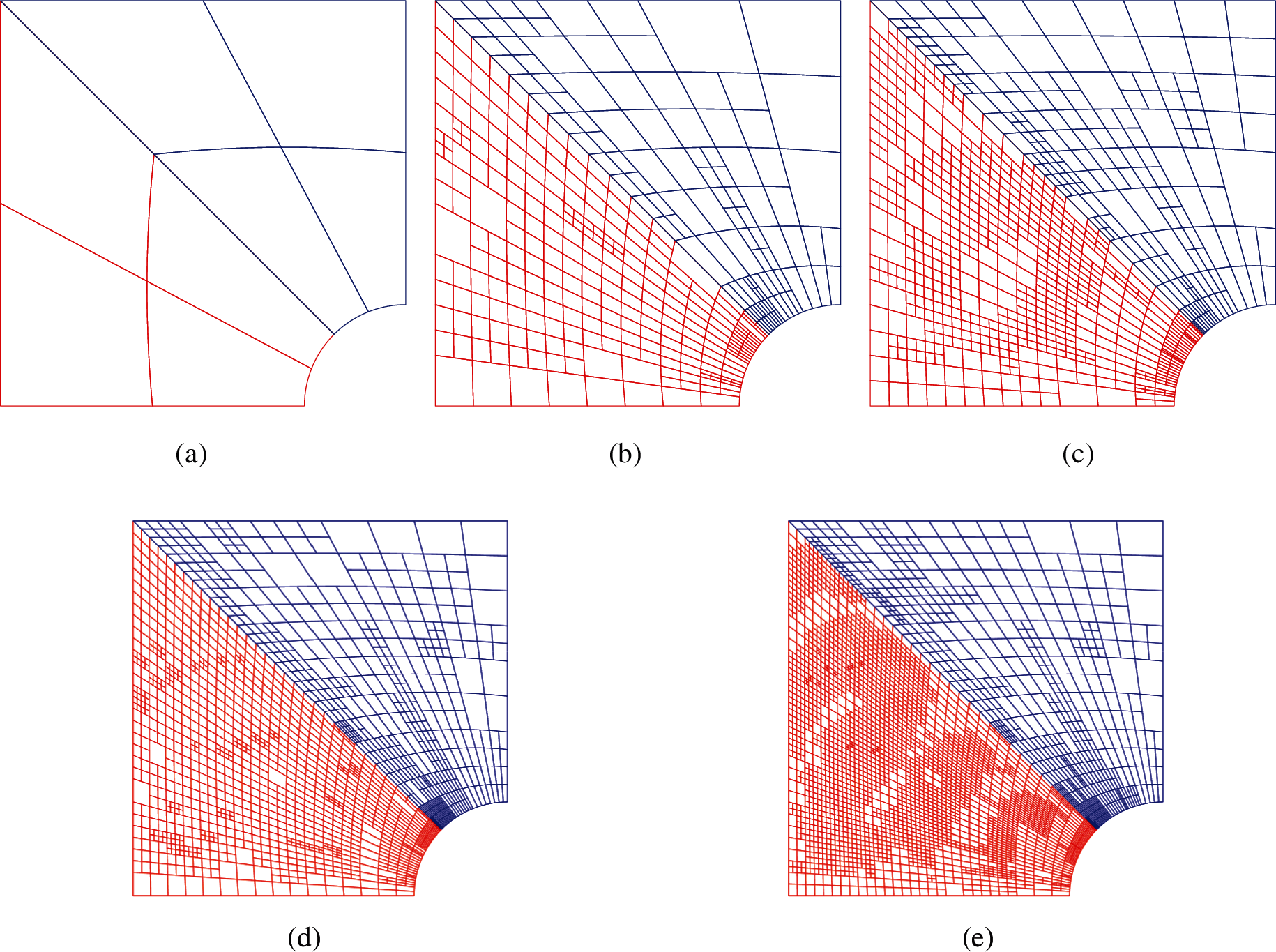Figure 12: Adaptive refinement process of 1–9th mode for vibration of platehole. Geometry-NURBS with order p1 = 1, p2 = 2; Solution Field-PHT with order q1 = 3, q2 = 3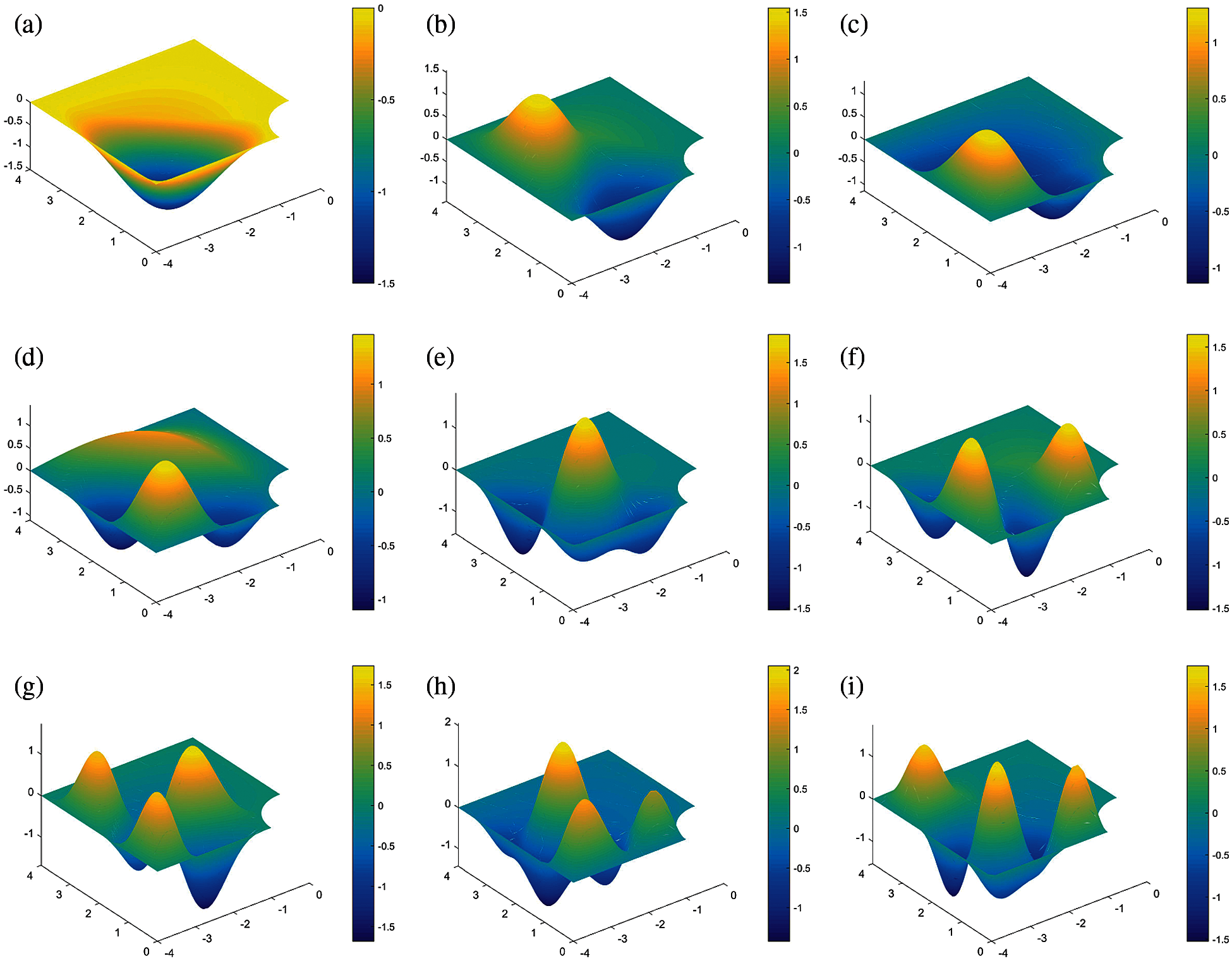Figure 13: Mode shapes 1–9th of platehole, where λNi is normalized natural frequency at mode i, calculated by λNi=λi/λ1,i=1,2,3n. (a) 1th mode,λN1=1; (b) 2th mode,λN2=2.03; (c) 3th mode,λN3=2.30; (d) 4th mode,λN4=3.12; (e) 5th mode,λN5=3.52; (f) 6th mode,λN6=4.20; (g) 7th mode,λN7=5.32; (h) 8th mode,λN8=5.45;(i) 9th mode,λN9=6.09Figure 14: (a) Comparisons of e1λ among refinement level of Le = 1, Le = 2, Le = 3; (b) error indicator of natural frequency of 1–9th mode during adaptive refinement process by sweeping modes; (c) contrast of relative error of natural frequency in energy norm at 1th mode between adaptive refinement and uniform refinement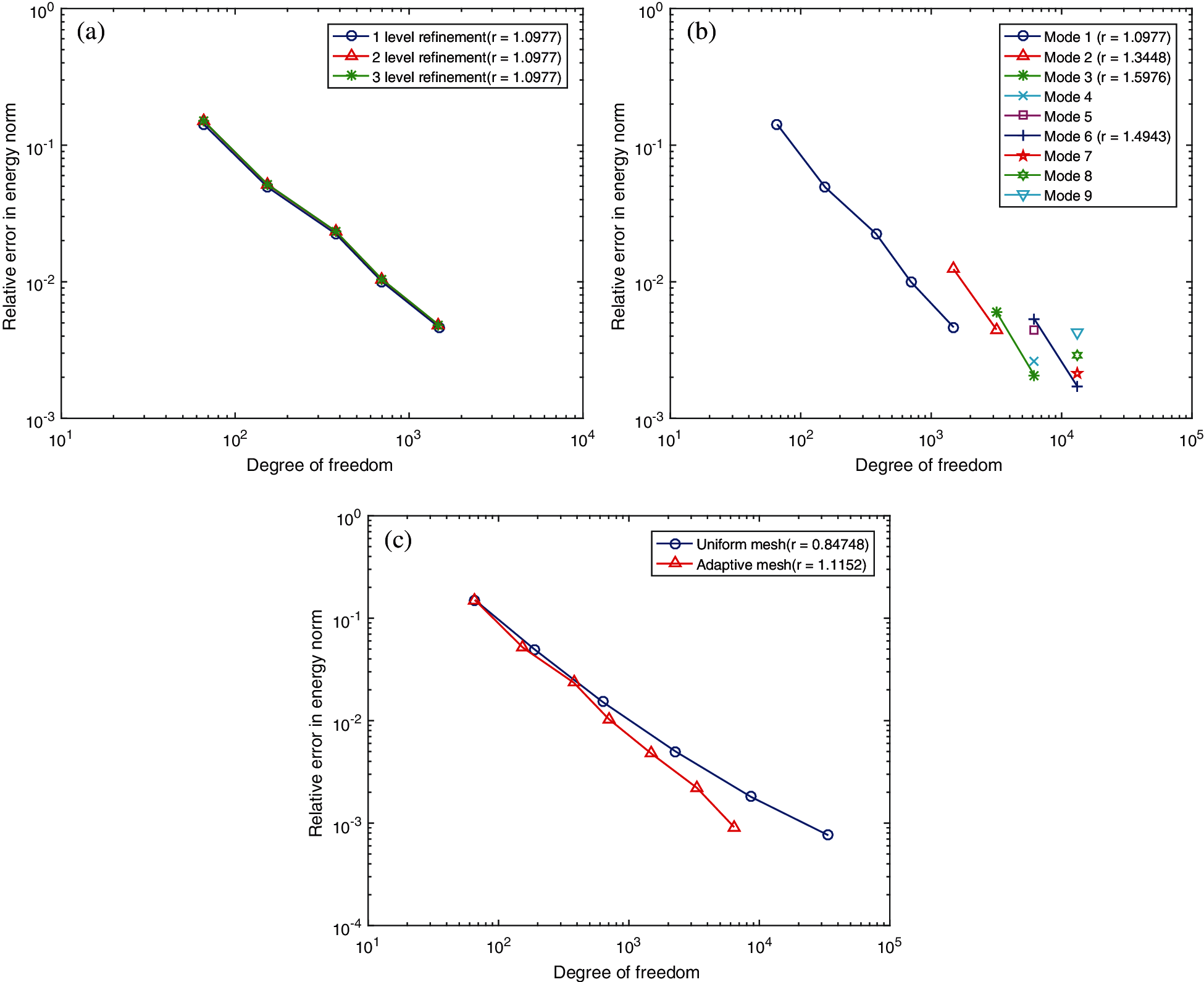Figure 15: (a) Comparisons of e1ϕ among refinement level of Le = 1, Le = 2, Le = 3; (b) error indicator of eigenvector of 1–9th mode during adaptive refinement process by sweeping modes; (c) contrast of relative error of eigenvector in energy norm between adaptive refinement and uniform refinement

6.4 Heterogeneous Lshaped-Bracket

The L-shape bracket is such a complex structure that it is divided into 18 Patches in Fig. 16b. Patches 1–4 are softer than other Patches that E1 − 4 = E, E5 − 18 = 100E = 109. Density ρ is 2700, and thickness h is 0.01 and Poisson's rate ν is 0.3. The whole boundary including the 4 holes is imposed to be simply supported except the right edge marked with red colour in Fig. 16a, where the boundary condition is assumed to be free. It is intended to make the vibration around Patch 4 is more fiercely than other parts. As a consequence, at the first 5 modes (1th–5th), the structural vibration and adaptive local refinement merge into Patches 1–4. When it comes to the 6th mode, the mode shape moves to the middle of structure (Patch 9, 10), seen in Fig. 18f. It induces the rise of the error (proved by plot of Mode 6 in Figs. 19b and 20b, respectively in this district and certainly the adaptive refinement will locate nearby, seen in Fig. 17e. After that, the modal shape moves back to Patches 1–4 and the adaptivity keeps going on in that zone. The convergence rate between adaptive refinement and uniform refinement are computed upon the basis of an approximate solution with 301,470 dof. Apparently, in Figs. 19c and 20, the convergence rate of adaptive refinement is much steeper (more than twice) than that of uniform refinement. This indicates the structural response is much local in this problem.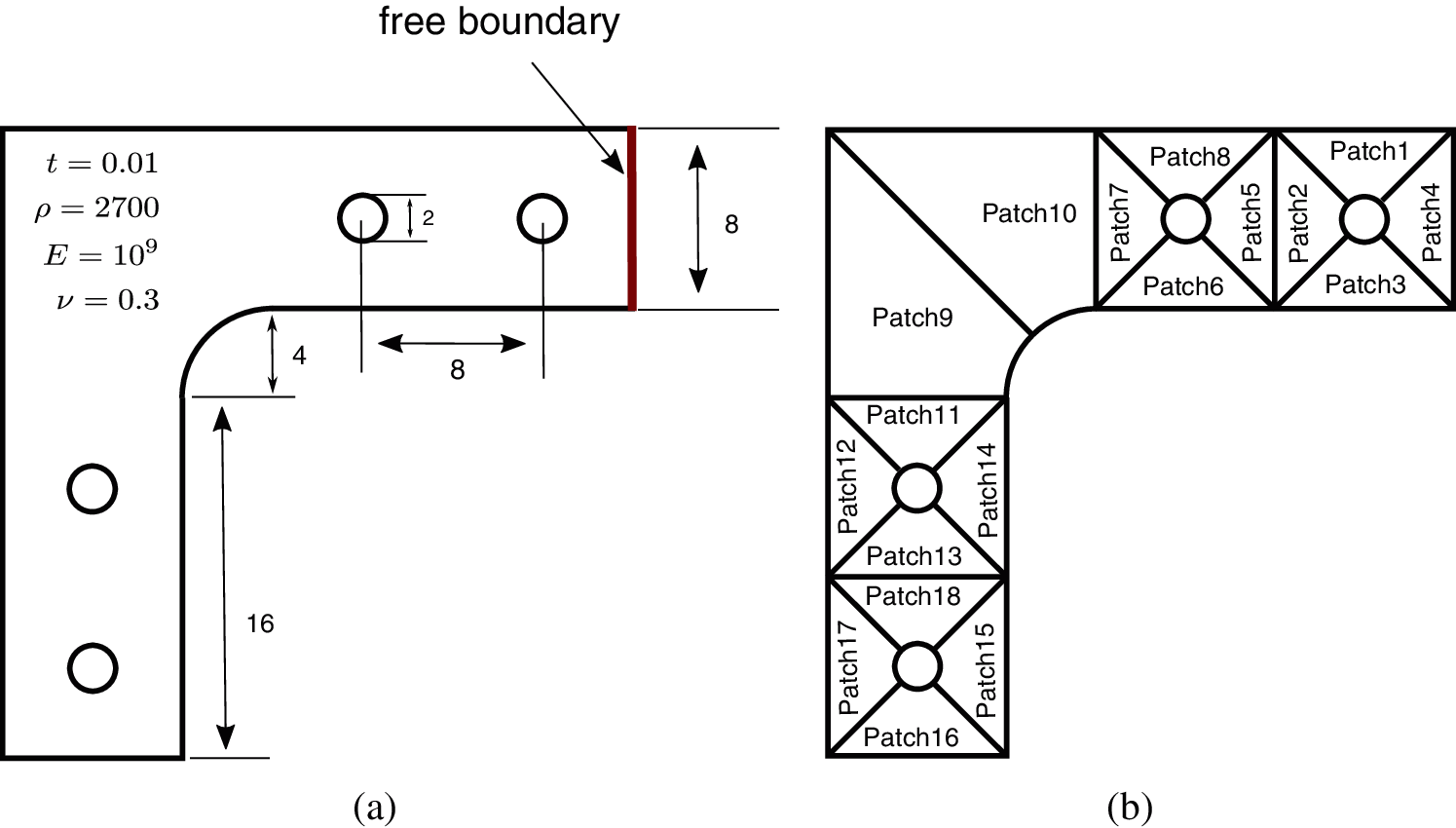Figure 16: A simply supported with right free boundary heterogeneous Lshaped-bracket: (a) geometric parameters and (b) discretization of patches, E1 − 4 = E, E5 − 18 = 100EFigure 17: Adaptive refinement process of 1–9th mode for vibration of Lshaped-bracket. Geometry-NURBS with order p1 = 1, p2 = 2; Solution Field-PHT with order q1 = 3, q2 = 3. (a) Initial mesh; (b) Adaptive mesh for 1th mode; (c) Adaptive mesh for 2th mode; (d) Adaptive mesh for 3, 4, 5th mode; (e) Adaptive mesh for 6, 7th mode; (f) Adaptive mesh for 8, 9th mode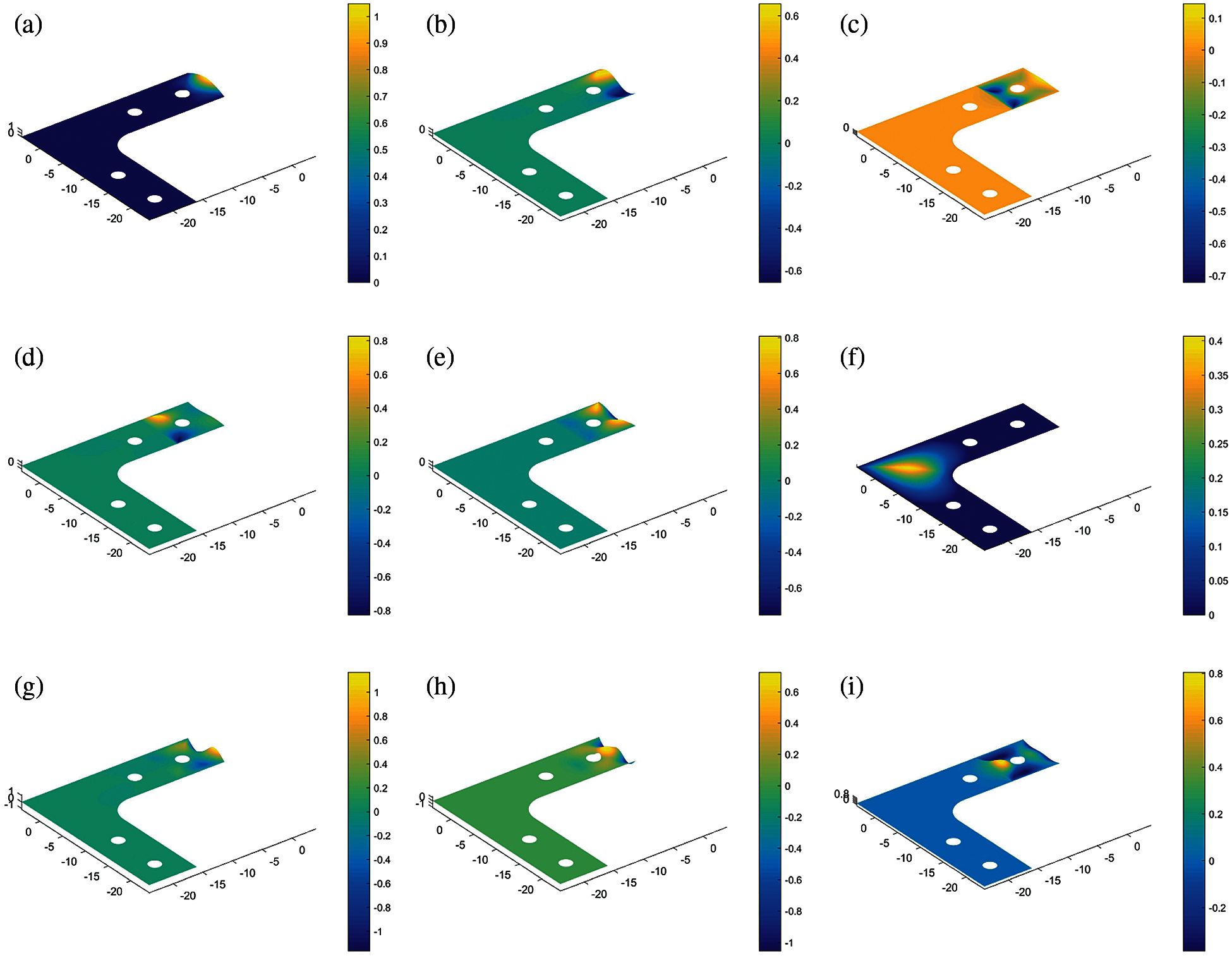Figure 18: Mode shapes 1–9th of Lshaped-bracket, where λNi is normalized natural frequency at mode i, calculated by λNi=λi/λ1,i=1,2,3n. (a) 1th mode,λN1=1; (b) 2th mode,λN2=2.79; (c) 3th mode,λN3=3.24; (d) 4th mode,λN4=3.52; (e) 5th mode,λN5=3.77; (f) 6th mode,λN6=4.30; (g) 7th mode,λN7=5.27; (h) 8th mode,λN8=6.23;(i) 9th mode,λN9=6.77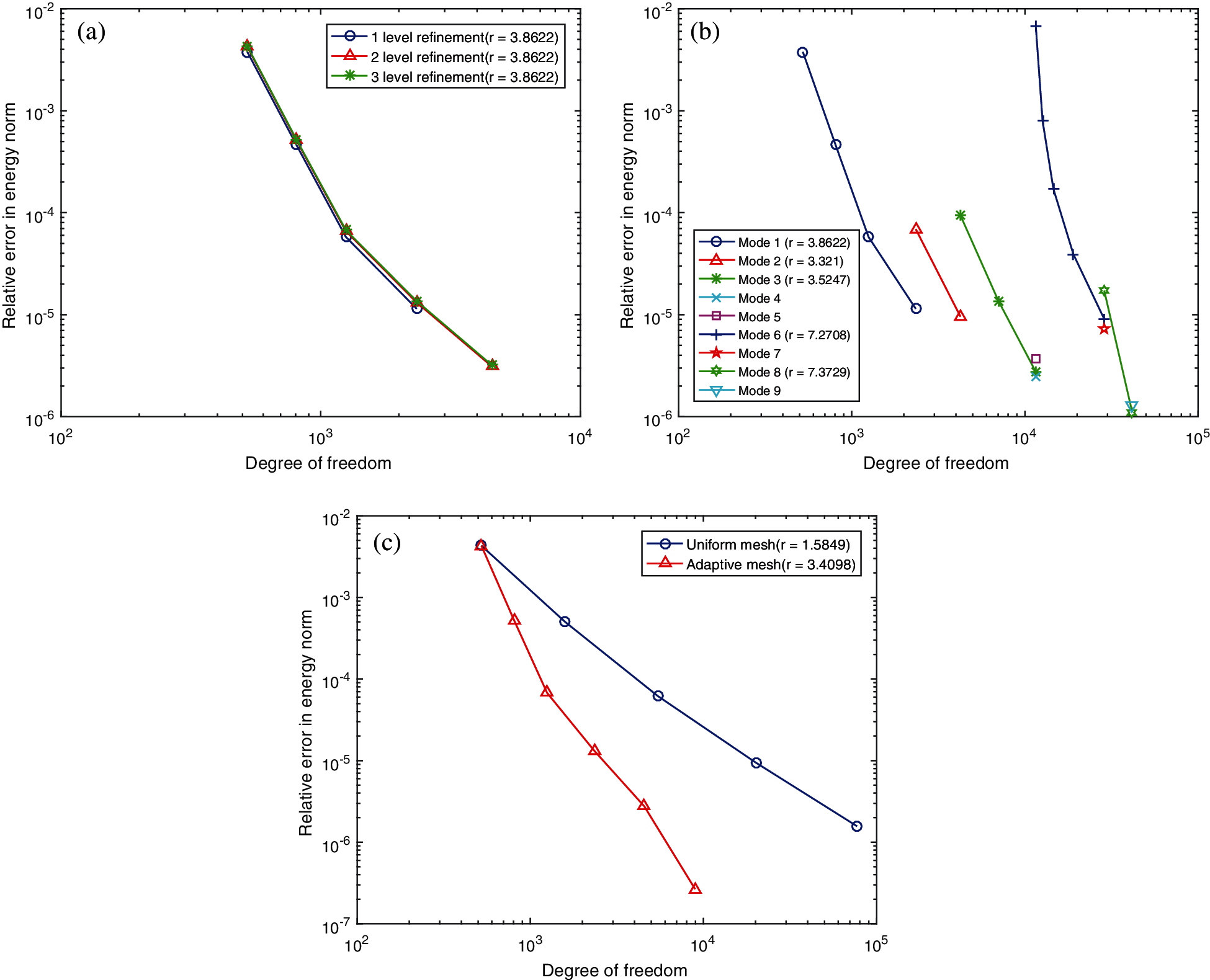Figure 19: (a) Comparisons of e1λ among refinement level of Le = 1, Le = 2, Le = 3; (b) error indicator of natural frequency of 1–15th mode during adaptive refinement process by sweeping modes; (c) contrast of relative error of natural frequency in energy norm between adaptive refinement and uniform refinement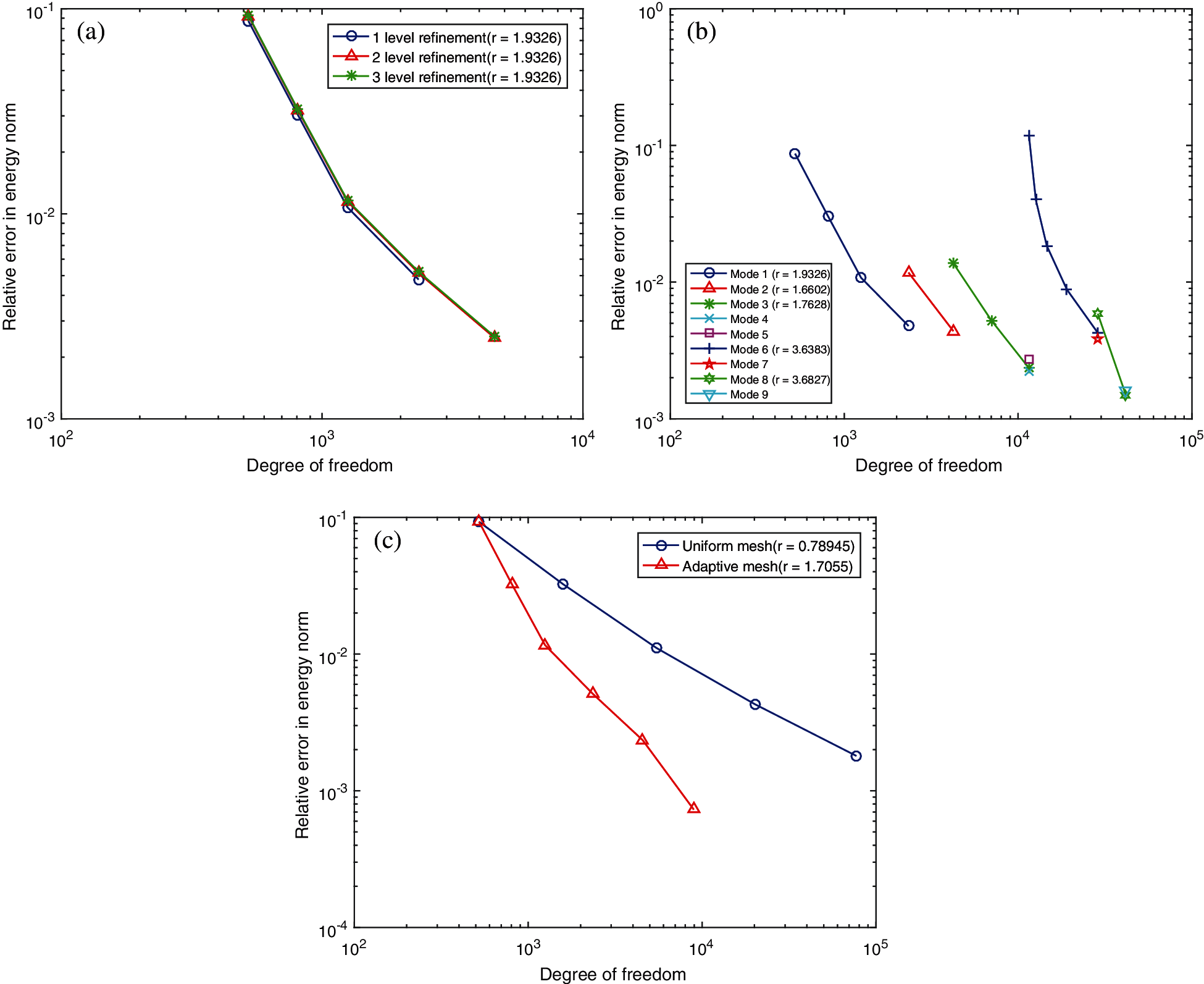Figure 20: (a) Comparisons of error indicator of eigenvector at 1th mode e1ϕ among refinement level of Le = 1, Le = 2, Le = 3; (b) error indicator of eigenvector of 1–15th mode during adaptive refinement process by sweeping modes; (c) contrast of relative error of eigenvector in energy norm between adaptive refinement and uniform refinement

7  Conclusion

In this article, based on our proposed adaptivity strategy in the framework of GIFT paradigm utilized for vibration of Reissner–Mindlin plate, it is developed to investigate the error-driven adaptivity for structural vibration of Kirchhoff plate. GIFT offers us the convenience to choose solution field independently from geometry so that the good accuracy can be achieved by better approximation in the solution field even though the geometric parameterization is not fairly designed as supported by the example in Section 6.1. Furthermore, it allows us to connect NURBS describing for geometry with PHT represented for solution field to reserve geometric precision and realize local refinement. Besides, when dealing with error estimation process, MAC scheme is helpful to locate the correspondence of modal vectors covering multiple eigenvectors between two different meshes. Our error indicator is proved to be reasonably chosen and the proposed adaptive strategy shows a better convergence rate than uniform refinement, supported by numerical tests in Sections 6.26.4.

We believe the proposed algorithm has potential to be practical in engineering problems. The developed method is available to precisely describe complex geometry of structure and simultaneously saves computational resource for given accuracy, especially for structures with local mechanical behaviour. As to the adaptivity of vibration by sweeping modes from low to high frequency, this adaptive strategy can be started from any mode or used to optimize some specific modes people are interested in. It would be productive to modal analysis for vibration problem. In the future, we intend to extend this method to 3D elasto-dynamics including the space-time problems.

Acknowledgement: This study was funded by Research Grant for 100 Talents of Guangxi Plan, The Starting Research Grant for High-Level Talents from Guangxi University, Generalized Isogeometric Analysis with Homogeniztion Theory for Soft Acoustic Metamaterials (20200312), Science and Technology Major Project of Guangxi Province (AA18118055), Guangxi Natural Science Foundation (2018JJB160052), and Application of Key technology in Building Construction of Prefabricated Steel Structure (BB30300105).

Funding Statement: This study was funded by Natural Science Foundation of China (Grant No. 12102095), Research grant for 100 Talents of Guangxi Plan, The Starting Research Grant for High-Level Talents from Guangxi University, Generalized Isogeometric Analysis with Homogeniztion Theory for Soft Acoustic Metamaterials (AD20159080), Science and Technology Major Project of Guangxi Province (AA18118055), Guangxi Natural Science Foundation (2018JJB160052), and Application of Key Technology in Building Construction of Prefabricated Steel Structure (BB30300105).

Conflicts of Interest: The authors declare that they have no conflicts of interest to report regarding the present study.

References

1.  1.  Hughes, T. J., Cottrell, J. A., Bazilevs, Y. (2005). Isogeometric analysis: CAD, finite elements, nurbs, exact geometry and mesh refinement. Computer Methods in Applied Mechanics and Engineering, 194(39), 4135–4195. DOI 10.1016/j.cma.2004.10.008.
2.  2.  Nguyen, V. P., Anitescu, C., Bordas, S. P., Rabczuk, T. (2015). Isogeometric analysis: An overview and computer implementation aspects. Mathematics and Computers in Simulation, 117, 89–116. DOI 10.1016/j.matcom.2015.05.008.
3.  3.  Lian, H., Kerfriden, P., Bordas, S. P. (2016). Implementation of regularized isogeometric boundary element methods for gradient-based shape optimization in two-dimensional linear elasticity. International Journal for Numerical Methods in Engineering, 106(12), 972–1017. DOI 10.1002/nme.5149.
4.  4.  Lian, H., Kerfriden, P., Bordas, S. (2017). Shape optimization directly from cad: An isogeometric boundary element approach using T-splines. Computer Methods in Applied Mechanics and Engineering, 317, 1–41. DOI 10.1016/j.cma.2016.11.012.
5.  5.  Chen, L., Lian, H., Liu, Z., Chen, H., Atroshchenko, E. et al. (2019). Structural shape optimization of three dimensional acoustic problems with isogeometric boundary element methods. Computer Methods in Applied Mechanics and Engineering, 355, 926–951. DOI 10.1016/j.cma.2019.06.012.
6.  6.  Cottrell, J. A., Reali, A., Bazilevs, Y., Hughes, T. J. (2006). Isogeometric analysis of structural vibrations. Computer Methods in Applied Mechanics and Engineering, 195(41–43), 5257–5296. DOI 10.1016/j.cma.2005.09.027.
7.  7.  Shojaee, S., Izadpanah, E., Valizadeh, N., Kiendl, J. (2012). Free vibration analysis of thin plates by using a NURBS-based isogeometric approach. Finite Elements in Analysis and Design, 61, 23–34. DOI 10.1016/j.finel.2012.06.005.
8.  8.  del Toro Llorens, A., Kiendl, J. (2021). An isogeometric finite element-boundary element approach for the vibration analysis of submerged thin-walled structures. Computers & Structures, 256, 106636. DOI 10.1016/j.compstruc.2021.106636.
9.  9.  Patton, A., Antolin, P., Dufour, J. E., Kiendl, J., Reali, A. (2021). Accurate equilibrium-based interlaminar stress recovery for isogeometric laminated composite kirchhoff plates. Composite Structures, 256, 112976. DOI 10.1016/j.compstruct.2020.112976.
10. 10. Sobota, P. M., Dornisch, W., Müller, R., Klinkel, S. (2017). Implicit dynamic analysis using an isogeometric reissner–mindlin shell formulation. International Journal for Numerical Methods in Engineering, 110(9), 803–825. DOI 10.1002/nme.5429.
11. 11. Thai, C. H., Nguyen-Xuan, H., Nguyen-Thanh, N., Le, T. H., Nguyen-Thoi, T. et al. (2012). Static, free vibration, and buckling analysis of laminated composite reissner–mindlin plates using nurbs-based isogeometric approach. International Journal for Numerical Methods in Engineering, 91(6), 571–603. DOI 10.1002/nme.4282.
12. 12. van Do, V. N., Lee, C. H. (2021). Isogeometric layerwise formulation for bending and free vibration analysis of laminated composite plates. Acta Mechanica, 232(4), 1329–1351. DOI 10.1007/s00707-020-02900-7.
13. 13. Shafei, E., Faroughi, S., Rabczuk, T. (2021). Nonlinear transient vibration of viscoelastic plates: A NURBS-based isogeometric HSDT approach. Computers & Mathematics with Applications, 84, 1–15. DOI 10.1016/j.camwa.2020.12.006.
14. 14. Chemin, A., Elguedj, T., Gravouil, A. (2015). Isogeometric local h-refinement strategy based on multigrids. Finite Elements in Analysis and Design, 100, 77–90. DOI 10.1016/j.finel.2015.02.007.
15. 15. Schillinger, D., Dede, L., Scott, M. A., Evans, J. A., Borden, M. J. et al. (2012). An isogeometric design-through-analysis methodology based on adaptive hierarchical refinement of NURBS, immersed boundary methods, and T-spline CAD surfaces. Computer Methods in Applied Mechanics and Engineering, 249, 116–150. DOI 10.1016/j.cma.2012.03.017.
16. 16. Sederberg, T. W., Cardon, D. L., Finnigan, G. T., North, N. S., Zheng, J. et al. (2004). T-spline simplification and local refinement. ACM Transactions on Graphics, 23(3), 276–283. DOI 10.1145/1015706.1015715.
17. 17. Bazilevs, Y., Calo, V. M., Cottrell, J. A., Evans, J. A., Hughes, T. J. R. et al. (2010). Isogeometric analysis using T-splines. Computer Methods in Applied Mechanics and Engineering, 199(5–8), 229–263. DOI 10.1016/j.cma.2009.02.036.
18. 18. Deng, J., Chen, F., Li, X., Hu, C., Tong, W. et al. (2008). Polynomial splines over hierarchical T-meshes. Graphical Models, 70(4), 76–86. DOI 10.1016/j.gmod.2008.03.001.
19. 19. Kang, H., Xu, J., Chen, F., Deng, J. (2015). A new basis for PHT-splines. Graphical Models, 82, 149–159. DOI 10.1016/j.gmod.2015.06.011.
20. 20. Forsey, D. R., Bartels, R. H. (1988). Hierarchical B-spline refinement. Proceedings of the 15th Annual Conference on Computer Graphics and Interactive Techniques, New York, USA.
21. 21. Vuong, A. V., Giannelli, C., Jüttler, B., Simeon, B. (2011). A hierarchical approach to adaptive local refinement in isogeometric analysis. Computer Methods in Applied Mechanics and Engineering, 200(49–52), 3554–3567. DOI 10.1016/j.cma.2011.09.004.
22. 22. Giannelli, C., Jüttler, B., Speleers, H. (2012). THB-splines: The truncated basis for hierarchical splines. Computer Aided Geometric Design, 29(7), 485–498. DOI 10.1016/j.cagd.2012.03.025.
23. 23. Giannelli, C., Jüttler, B., Kleiss, S., Mantzaflaris, A., Simeon, B. et al. (2016). THB-splines: An effective mathematical technology for adaptive refinement in geometric design and isogeometric analysis. Computer Methods in Applied Mechanics and Engineering, 299, 337–365. DOI 10.1016/j.cma.2015.11.002.
24. 24. Nguyen-Thanh, N., Nguyen-Xuan, H., Bordas, S. P. A., Rabczuk, T. (2011). Isogeometric analysis using polynomial splines over hierarchical t-meshes for two-dimensional elastic solids. Computer Methods in Applied Mechanics and Engineering, 200(21–22), 1892–1908. DOI 10.1016/j.cma.2011.01.018.
25. 25. Nguyen-Thanh, N., Muthu, J., Zhuang, X., Rabczuk, T. (2014). An adaptive three-dimensional rht-splines formulation in linear elasto-statics and elasto-dynamics. Computational Mechanics, 53(2), 369–385. DOI 10.1007/s00466-013-0914-z.
26. 26. Atroshchenko, E., Tomar, S., Xu, G., Bordas, S. P. (2018). Weakening the tight coupling between geometry and simulation in isogeometric analysis: From sub-and super-geometric analysis to Geometry-Independent Field approximaTion (GIFT). International Journal for Numerical Methods in Engineering, 114(10), 1131–1159. DOI 10.1002/nme.5778.
27. 27. Yu, P., Anitescu, C., Tomar, S., Bordas, S. P. A., Kerfriden, P. (2018). Adaptive isogeometric analysis for plate vibrations: An efficient approach of local refinement based on hierarchical a posteriori error estimation. Computer Methods in Applied Mechanics and Engineering, 342, 251–286. DOI 10.1016/j.cma.2018.08.010.
28. 28. Liu, G. (2010). Meshfree Methods: Moving Beyond the Finite Element Method, CRC Press; Boca Raton.
29. 29. Liu, Y., Hon, Y., Liew, K. (2006). A meshfree hermite-type radial point interpolation method for kirchhoff plate problems. International Journal for Numerical Methods in Engineering, 66(7), 1153–1178. DOI 10.1002/(ISSN)1097-0207.
30. 30. Fernández-Méndez, S., Huerta, A. (2004). Imposing essential boundary conditions in mesh-free methods. Computer Methods in Applied Mechanics and Engineering, 193(12), 1257–1275. DOI 10.1016/j.cma.2003.12.019.
31. 31. Kiendl, J., Bazilevs, Y., Hsu, M. C., Wüchner, R., Bletzinger, K. U. (2010). The bending strip method for isogeometric analysis of kirchhoff–love shell structures comprised of multiple patches. Computer Methods in Applied Mechanics and Engineering, 199(37), 2403–2416. DOI 10.1016/j.cma.2010.03.029.
32. 32. Allemang, R. J. (2003). The modal assurance criterion–twenty years of use and abuse. Sound and Vibration, 37(8), 14–23.
33. 33. Pastor, M., Binda, M., Hararik, T. (2012). Modal assurance criterion. Procedia Engineering, 48, 543–548. DOI 10.1016/j.proeng.2012.09.551.
34. 34. Leissa, A. W. (1969). Vibration of plates, vol. 160. Scientific and Technical Information Division, National Aeronautics and Space Administration.This work is licensed under a Creative Commons Attribution 4.0 International License, which permits unrestricted use, distribution, and reproduction in any medium, provided the original work is properly cited.